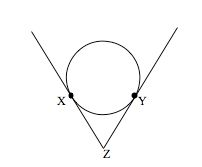# ACT and SAT Advanced Geometry

Advanced geometry questions on the ACT and SAT can range from triangle, circles, and cubes to more advanced questions like inscribed shapes. If you need to brush up on the basics check out this post. If not, let’s get ready for some advanced questions!

### Basic Geometry Concepts and Formulas

1. a² + b² = c², but only when a right triangle. If you don’t know it’s a right triangle, Pythagorean theorem does not apply!
2. Common special right triangles include 3-4-5, 5-12-13, 8-15-17, 7-24-25 (and their multiples.)
3. 45-45-90 triangles are ALWAYS in the ratio 1:1:√2
4. 30-60-90 triangle are ALWAYS in the ratio 1:√3:2
5. Angles and opposite sides are in the same relative size order, but are NOT proportional.

Let’s continue with a standard diagram in which we have an equilateral triangle inscribed in a circle, which is inscribed in a square.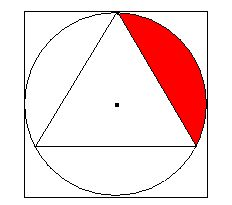The center point of all three figures (triangle, circle, square) are all the same, but this is ONLY true if the triangle is equilateral  (all the sides are the same length). Therefore, if given ANY piece of information about the circle, square or triangle, we can derive the rest. We draw a perpendicular line from the center to the side of the triangle.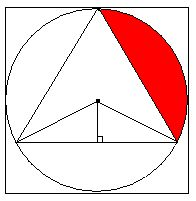Note that the hypotenuses of the smaller triangles are equal to the radius of the circle. We also know that the smaller triangles are each 30-60-90 because you are taking the 120-degree internal angle from the circle’s center and cutting it in two. Here are your basic conversions:
r = ½d = ½s, where s is the side of the square.
The sides of the 30-60-90 triangles become ½r : (r√3)/2 : r respectively
The side of the equilateral triangle becomes 2*(r√3)/2 = r√3
If given the area of the square, we should be able to derive essentially any other information.

### Area of an Equilateral Triangle

The area of an equilateral triangle equals (s²√3)/4. Memorize this. It will save you the time of drawing a 30-60-90 triangle, solving for the base, finding the height, multiplying and dividing by 2. That was long to write, imagine how long it takes to do!
If  the area of the square = 64 and we needed to find the area of the triangle, we just use the conversions above:
d = 8
r = 4
side of triangle = 4√3
Area of triangle = [(4√3)²√3]/4 = 16*3*√3 / 4 = 4*3*√3 = 12√3

### Angle Relationships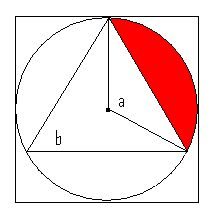Another important rule is that the interior angle created from of two radii extending to the outside of the circle is exactly twice the measure of any angle on the circle extending to those same points. In the image above, 2b = a.
Let’s take a look at this practice question: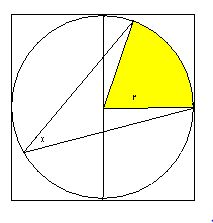In the figure above, a circle is inscribed in a square. If the perimeter of the square is 32 and x=35, what is the area of the shaded region?
We can determine r by knowing that the length of the square’s side. If s = 32/4 = 8, then d = 8 and r = 4. But we still need to know the interior angle.  Since x=35, we know that the interior angle of the shaded region is 2(35) = 70. So A(shaded) = (2x/360) * πr² = 70/360 * 16π = 28π/9

### Arc Lengths and Sector Areas

One of the key things to remember with circles is that once you know one piece of information, you know everything about the circle itself. Additional angles and lengths inside are not always so simple, but it is possible to convert circumferences to areas, to radii and diameters without intermediate steps.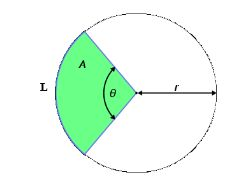Arc Lengths (portions of perimeters) and Sectors (pie slices) seem more complicated than they really are. Both relate directly to the internal angle at the circle’s center, represented by θ in this diagram. Here are the equations:
Sector Area = A = θ/360 * πr²
Arc Length = L = θ/360 * 2πr
θ in these formulas refer to the angle measure in degrees, not radians. If you’re using your calculator, make sure that your calculator is in the right mode.
All we are doing is finding the fraction of the circle and applying it to either the area or circumference formula, respectively. The pie is always a certain fraction of the circle, meaning the area of the pie and the arc length of the pie is also the same fraction of the circle’s area and circumference respectively.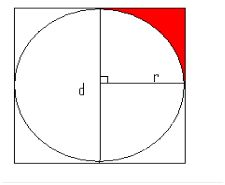In the above diagram, a square is inscribed in a circle, which is inscribed in another square. If the area of the larger square is 64 and the area of the green region is 4, which is larger, the green region or the yellow region?
The information we have is:
A(large square) = 64
s(large square) = 8
diameter = 8
r = 4
First, recognize that the figure is symmetrical. So while we may not explicitly be given an angle to find the sector area (not drawn) in which the yellow region resides, we do know its measure. The diagonals of a square intersect at a right angle, so we can deduce that the sector including the yellow region is 1/4 of the area of the circle. Since we know the radius…
A(large sector) = 1/4 * 16π = 4π
To find the yellow region itself, we must subtract the imaginary (not drawn) triangle from 4π. (Note that this imaginary triangle will be twice the green triangle.)
A(imaginary triangle) = 1/2 * r * r = 1/2 * 4 * 4 = 8
A(Yellow Region) = 4π – 8
The area of the green region can be found in two ways. Either we can see that it’s simply one-half of the 8 we just found, OR we can find both sides of the green triangle with the common 45-45-90 1:1:√2 formula. With a hypotenuse of 4, we derive 2√2 for each side, which yields an area of 4.